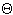Hi there. A long and ultimately fruitless search through Google has led me to you. I'm wanting to build a series of architectural models of different roman and medieval buildings out of cardboard. Once I have perfected the models I want to print them out on card stock so that school kids (or anyone else) can make the buildings. A feature of many of these models is the cross or groin vault (a perpendicular intersection of two barrel vaults). A single barrel vault is easy to imagine as a plane (a rectangular piece of cardboard) that will be folded into a semi-circular arch. The intersection of a second barrel vault and this one is presenting me with problems. The second plane needs to have an ellipse cut into it so that when it is folded into the arch, it will mate up with the curve of the first barrel vault. Given that the two pieces of card have identical widths (and therefore identical arcs in cross section) is there a way to calculate the ellipse that needs to be cut so that it can be cut before the second arch is folded? Any help you might be able to give would be greatly appreciated Kind regards Neal Hi Neal, By symmetry you need to do is find the intersection of a cylinder and a plane with inclination 45 degrees. Cylindrical coordinates (r,, z) give a nice development. Equation of cylinder is r=r0. Equation of plane is z = x, i.e., z = r cos. Intersection of two surfaces is (r0,, r0 cos). Already parameterized by theta, so unroll it and voila, you have a graph of one complete phase of cosine. :-) For your application, you only need a quarter of the unrolled cylinder for half of the upper half of a tunnel, so you need cosine from its minimum to the point where it crosses the axis and then back down again, i.e. half a period of -r0 |cos|. If you're cutting the thing out of strips of paper, the amplitude of the |cos| function should be  width of paper/. I created a template for one vault with a radius of 5 cm. If you print this make sure you turn off all scaling. The width of the template should be approximately 15 cm. If you want a different radius then let us know. Ed